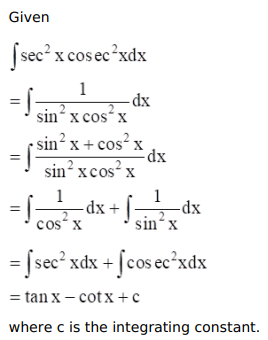# Mark against the correct answer in each of the following:

Question:

Mark $(\sqrt{)}$ against the correct answer in each of the following:

$\int \sec ^{2} x \operatorname{cosec}^{2} x d x=?$

A. $\tan x-\cot x+C$

B. $\tan x+\cot x+C$

C. $-\tan x+\cot x+C$

D. none of these

Solution: